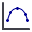Expert Modelling: Hands-on help from Rocscience Engineers. Register Here

# Total Kinetic Energy

To plot total kinetic energy:

1. Select Graph Data on Slopefrom the Graphs menu or from the toolbar.
2. The Graph Data on Slope dialog will appear.
3. Select Total Kinetic Energy as the variable to plot using the Data drop-down menu.
4. Select the value to plot: user-specified percentile, max (envelope), or mean.
5. Specify the number of Horizontal Locations used to create the graph.
6. Select the Paths to Plot using the drop-down menu. Choose from All Rock Paths or Paths with Selected Rocks.
7. If you opted to plot Paths with Selected Rocks, choose the seeder you want to plot.
8. Click Plot Data.

The horizontal axis of the Total Kinetic Energy graph is the x-coordinate of the slope and the vertical axis is the max, average, or specified percentile total kinetic energy at that location.

The location of each vertex on the graph (where the total kinetic energy is sampled) is determined by the number of Horizontal Locations. The width of each bin is calculated by dividing the width of the slope (maximum x-coordinate – minimum x-coordinate of the slope) by the number of Horizontal Locations (a.k.a. intervals, divisions). You specify the number of Horizontal Locations used to create the graph in the Graph Data on Slope dialog before you plot the graph.

#### Notes:

• Total Kinetic Energy = Rotational + Translational Energy
• The number of Horizontal Locations cannot be changed in the Chart Properties dialog. Make sure you specify the appropriate number of Horizontal Locations in the Graph Data on Slope dialog.
• If the graph appears to be "blocky" or changes considerably when more falling rocks are analyzed, you should probably increase the number of sampling intervals (Horizontal Locations).
• It may be useful to use a data collector or distribution graph to determine the distribution of energies at a specific location, once you have used this graph to narrow down a location of interest.
• The graph appearance can be customized in the Chart Properties dialog, available from the right-click menu.